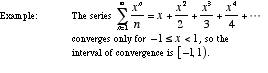index: click on a letter A B C D E F G H I J K L M N O P Q R S T U V W X Y Z A to Z index index: subject areas numbers & symbols sets, logic, proofs geometry algebra trigonometry advanced algebra & pre-calculus calculus advanced topics probability & statistics real world applications multimedia entrieswww.mathwords.com about mathwords website feedback

 Interval of Convergence For a power series in one variable, the set of values of the variable for which the series converges. The interval of convergence may be as small as a single point or as large as the set of all real numbers.See also